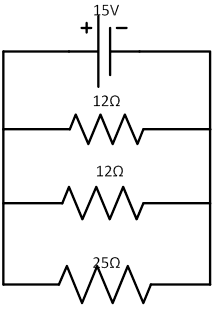### Sample ProblemWhat is the equivalent resistance in the circuit?

#### Solution

1/Req = 1/R1 + 1/R2 + 1/R3 = 1/12 + 1/12 + 1/25 = 31/150

Req = 1 ÷31/150 = 4.8 Ω